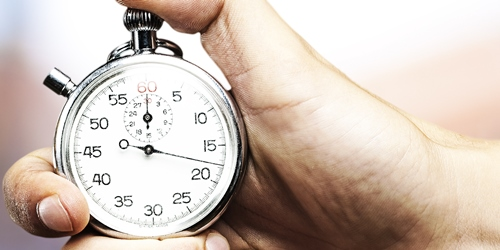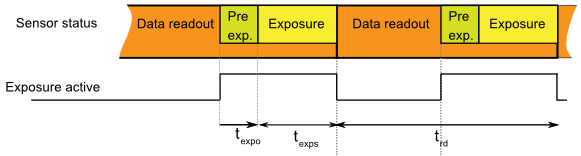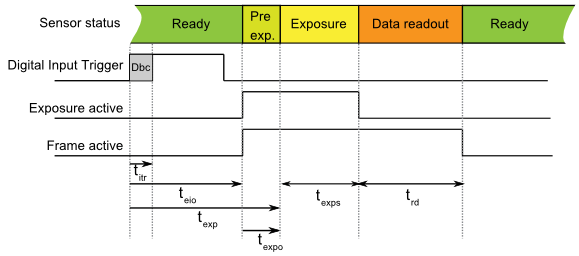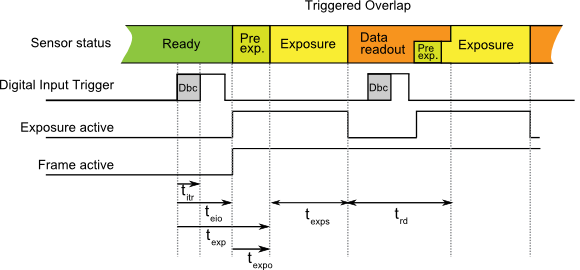Support» Knowledge Base » Sensors Signals Timing

# Sensors Signals Timing¶` `
Each sensor has different timing.
The real timing depends on multiple parameters:
` `

• Exposure Time (ET). This time is defined by xiAPI by (XI_PRM_EXPOSURE)
• Data Readout Time (t rd). It depends on:
• Sensor bit depth
• Binning or Skipping selected
• Region of Interest (ROI) used
• Sensor clock which depends on Transfer speed (MB/s)
• Amount of data transferred (MB) - depends on image resolution and number of bits transferred per pixel (BPP)
• Channel count used for data read out

` `

## Calculators - frame rate¶

In order to simplify the calculation of frame-rate or other parameters - use one of the following calculators based on camera sensor type:
` `
` `

Camera speed calculator

Camera_Performance_Calculator.xlsm (deprecated)

For:

• CMOSIS (AMS Osram): CMV12000, CMV20000, CMV50000
• Sony: IMX174, IMX250, IMX252, IMX253, IMX255
• Sony Pregius S: IMX540, IMX541, IMX542, IMX530, IMX531, IMX532
• Sony Medium Format: IMX455, IMX461, IMX411
• Gpixel: GSENSE2020, GSENSE2020-BSI and GSENSE6060 BSI (Backside illuminated version), GSENSE5130
• Gpixel: GMAX3265, GMAX0505
• ON Semiconductor: AR1820HS
• Luxima: LUX13HS, LUX19HS, LUX160

` `

FPS_calculator_CMV.xlsx

For:

• CMOSIS (AMS): CMV4000, CMV2000, CMV300

` `

## Free-Run mode (no trigger)¶

Each image exposition is started automatically when possible.
This mode gives the highest fps (frames per second) that is possible on the sensor.

### Exposure Overlapped with Data Readout Mode¶

This Overlapped mode gives the highest number of frames per second.
` `
` `Sensor timing in Exposure Overlapped with Data Readout Mode

` `
In this mode, the timing depends on the minimum Exposure Time and Data Readout Time.

` `
` `

## Triggered mode without overlap¶

Each image exposition is triggered with the input trigger signal.
This mode gives a lower fps value compared to Free-Run mode.

### Exposure Not Overlapped with Data Readout Mode¶

Most sensors use this mode when the input trigger is active.
This mode gives a lower fps value than Exposure Overlapped with Data Readout mode.
` `
` `Sensor timing in Exposure Not Overlapped with Data Readout Mode

` `
In this mode, the timing depends on the sum of:
` `

• Input transition time (t itr) depends on:
• Digital Input Delay - time for changing the internal circuit to active state. It is constant for each camera model.
• Input Debouncing Time - time for stabilizing uneven input signals (e.g. from mechanical switches). This time can be set using xiAPI with parameters XI_PRM_DEBOUNCE_EN and XI_PRM_DEBOUNCE_T0 on some cameras. Default 0.
• Exposure time (see ET above).
• Data Readout time (see t rd above)

` `

### Table1: Typical times for selected camera models¶

 DownS t itr [µs] t exp [µs] t eio[µs] t expo[µs] t rd[µs] Notes MU9 models: any 200+ xiQ/MQ models: any 1.4 29 19/238 10 400+16.4*BWF*LC N1, N2 MD models: any 2 <3 26/165 1 - N3 any <70ns <1 <1 <70ns - N4

` `

Note N1: V(Input)=15V
Note N2: x in model name means all available models (M, C, R)
Note N3: Optoisolated Input and output are used. Input rising edge. For falling edge the delay is plus 48us
Note N4: Isolated High Speed Digital Lines (5V TTL only)

Table1 legend:
DownS = Current camera DownSampling (XI_PRM_DOWNSAMPLING)
t eio = Trigger (Digital Input) to Strobe (Digital Output) (on some models is listed: Off->On change / On->Off change)
t exp = Strobe (Sensor) to Digital Output (on some models is listed: Off->On change / On->Off change)
t expo = Start of exposition to Exposure Active Digital Output
LC = Current Line Count (XI_PRM_ HEIGHT)
BWF = Bandwidth Factor for maximum bandwith this is 1 when the bandwith will be lower BWF will rise (TBD)
t exps = Current Exposure Time set (XI_PRM_EXPOSURE)
Conditions: XI_PRM_DEBOUNCE_EN=0 (off).
` `

### Minimum trigger period (Ttrig_min)¶

The Minimum trigger period can be calculated using the following formula:
• t trig_min = t exp + t exps + t rd
` `
Example for MQ013MG-E2, Exposure time = 500us, image = 500 pixels width x 200 pixels height.
• t trig_min = 29µs + 500µs + 400µs + 16.6µs * 200lines = 4249µs

` `
` `

## Triggered mode with overlap¶

Several sensors are capable to trigger exposure in overlap mode, so it is capable to reach the same fps value as in free run mode.

When the trigger period is longer than exposure and readout the signal waveform will look similar to Trigger mode without overlap.

However, when the trigger period will decrease the sensor will expose images in overlap mode.
In this case frame active signal will be all the time active.
` `
` `Sensor timing in Exposure Overlapped with Data Readout Mode

` `

The maximum FPS can be calculated using FPS_calculator_CMV.xlsx .

` `
For timing description please see the previous paragraph Triggered mode without overlap

### Table2: Typical times for selected camera models¶

 Camera Model DownS t itr [µs] t exp [µs] t eio[µs] t expo[µs] t rd[µs] t fot[µs] Notes MQ042xG-CM any 1.4 10 11/224 0 (64.5+5.375*LC)*BWF 64.5 N1,N2 MQ022xG-CM any 1.4 10 11/224 0 (37.625+5.375*LC)*BWF 37.625 N1,N2 MQ003xG-CM any 1.4 10 11/224 0 (92.8 + 3.78 * LC)*BWF 92.8 N1,N2, N3 MQ013xG-ONV any 1.4 <2 11/224 0 (23*W+3300)/BW+(7.66*W+1106)*LC/BW N1,N2,N4 MQ013xG-ON any 1.4 <2 11/224 0 TBD N1,N2

` `

Note N1: V(Input) = 15V
Note N2: 8 bit per pixel maximum bandwidth (TBD)
Note N3: Exposure is delayed for short exposure times. If (t exps < t rd) then t eio = t eio + (t rd-t exp) approx.
Note N4: VITA1300

Table2 legend:

DownS = Current camera DownSampling (XI_PRM_DOWNSAMPLING)
t eio = Trigger (Digital Input) to Strobe (Digital Output) (on some models is listed: Off->On change / On->Off change)
t exp = Strobe (Sensor) to Digital Output (on some models is listed: Off->On change / On->Off change)
t expo = Start of exposition to Exposure Active Digital Output
t exps = Current Exposure Time set (XI_PRM_EXPOSURE)
W = Image width(XI_PRM_WIDTH)
LC = Current Line Count (XI_PRM_ HEIGHT)
BW = Bandwidth in Mbit/s
BWF = Bandwidth Factor see table below
Conditions: XI_PRM_DEBOUNCE_EN=0 (off).

` `

### Bandwidth factor¶

Bandwidth factor is a number reflecting the ratio between the maximum pixel clock frequency and the current pixel clock frequency calculated from the Bandwidth Limit.

BWF = F max / F limit

Where F max is the maximum possible pixel clock frequency for the given sensor (in MHz) and F limit is the current pixel clock frequency which depends on the bandwidth limit parameter set in the API (XI_PRM_LIMIT_BANDWIDTH). F limit is set in 1MHz steps and can not go lower than F min.

### Table3: pixel clock info¶

 Camera Model F max [MHz] F min [MHz] F limit [MHz] Note MQ042xG-CM MQ022xG-CM 48 5 ((129*BWL)/(1024*BPP))*((12+LC)/LC)*(W max/W) N1 MQ003xG-CM 43 38 ((325*BWL*)/(316224*BPP))*(13+LC/2)*(W max/W)

` `

Note N1: Sensor is capable to limit the number of channels for readout.
The presented formula is valid down to half of the maximum bandwidth. Will be updated.

Table3 legend:
BPP - number of bits per pixel of the transport pixel format
BWL - currently set bandwidth limit in Mbit/s
W - image width
W max - maximum possible image width

` `

### Minimum trigger period (t trig_min )¶

The Minimum trigger period can be calculated using the following formula:
• t trig_min = t exp + t exps (When exposure time is longer than readout time)
• t trig_min = t rd (When the exposure time is significantly shorter than readout time)
• t trig_min = t exps + t fot (When exposure is smaller than readout time but the difference is less than t fot )
Example for MQ022xG-CM, Exposure time = 500us, image = 2048 pixels width x 1088 pixels height with maximum bandwidth and 1 byte per pixel.
• t trig_min = 10µs + (37.625+5.375*1088lines) * 1 = 5895µs

` `
` `